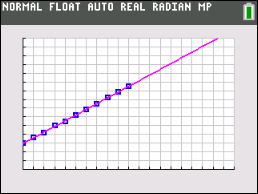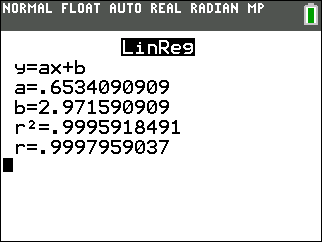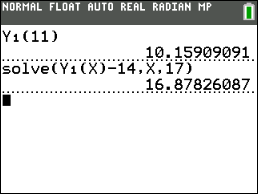••• ##### Device
• TI-83 Plus Family
• TI-84 Plus
• TI-84 Plus Silver Edition
•TI-84 Plus C Silver Edition
•TI-84 Plus CE
• ##### Report an Issue

Middle Grades Math: The Marble Experiment
by Texas Intruments#### Overview

Students determine, by examining a graph, if a data set has a positive or negative correlation coefficient.

#### Key Steps

•Students will graph a scatterplot of data and determine a linear function that describes the data.

•Students will determine the equation of a line of best fit to analyze and make predictions about data.Students will graph a scatterplot of data and determine a linear function that describes the data.

•Students will interpret the real-world significance of the slope and y-intercept of a linear model.